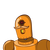# the ticket of 2 adult and 1 child is 3900. 3 adult and 2 children is 6300. find the fair and seat cost​

the ticket of 2 adult and 1 child is 3900. 3 adult and 2 children is 6300. find the fair and seat cost​

### 1 thought on “the ticket of 2 adult and 1 child is 3900. 3 adult and 2 children is 6300. find the fair and seat cost​”

1.Step-by-step explanation:

y- cost for a child

2x + y = 3900

3x + 2y = 6300

Multiply 1st equation by 2

4x + 2y = 7800

( – )

3x + 2y = 6300

———————–

x + 0y = 1500

————————

x = 1500

2x + y = 3900

y = 3900 – 2x

sub x=1500

y = 3900 – 2 (1500)

y = 3900 – 3000

y = 900

cost for an adult = 1500

coat for a child = 900